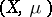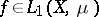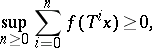# Maximal ergodic theorem

(diff) ← Older revision | Latest revision (diff) | Newer revision → (diff)

Ifis an endomorphism of a measure space, ifand ifis the set offor whichthenThe maximal ergodic theorem is due to K. Yosida and S. Kakutani , who showed that it can play a central role in the proof of the Birkhoff ergodic theorem (G.D. Birkhoff himself, instead of the maximal ergodic theorem, used somewhat different arguments). In later proofs of generalizations of Birkhoff's theorem (and also in related questions on the decomposition of the phase space into conservative and dissipative parts under conditions such that these generalizations make sense) a generalized maximal ergodic theorem is used in a similar way. There is a generalization of the maximal ergodic theorem due to E. Hopf and a simple proof of this generalization was given by A. García (see ). See also  and the references in Birkhoff ergodic theorem.

How to Cite This Entry:
Maximal ergodic theorem. Encyclopedia of Mathematics. URL: http://encyclopediaofmath.org/index.php?title=Maximal_ergodic_theorem&oldid=17718
This article was adapted from an original article by D.V. Anosov (originator), which appeared in Encyclopedia of Mathematics - ISBN 1402006098. See original article